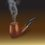# Limit Superior and Limit Inferior of a Bounded Real Sequence

Every undergraduate student who has done a basic course on real analysis learns something about limit points of a real sequence. We know that a bounded real sequence has at least one limit point. And we also know that the set of all limit points of a sequence (which is obviously bounded) has its supremum and infimum within itself. That is to say, the supremum and infimum of the set of all limit points of the bounded sequence are themselves limit points of the sequence. The supremum is called "limit supremum" and the infimum is called "limit infimum" of the sequence. This is how many textbooks on analysis define the two terms. However other textbooks define them as:

$\lim\sup \{x_n\} = \inf_{n \ge 0} \sup_{m \ge n} \{x_m\} = \inf \{ \sup \{ x_m : m\ge n\} : n\ge 0\}$

$\lim\inf \{x_n\} = \sup_{n \ge 0} \inf_{m \ge n} \{x_m\} = \sup \{ \inf \{ x_m : m\ge n\} : n\ge 0\}$

Try to establish an equivalence between these two definitions. When I learnt these, I failed to find such an attempt to establish the aforesaid equivalence even in good texts on the subject. The proof, as I had to chalk myself out at that time, was not too difficult, but was a cumbersome one and required considerable mental gymnastics. So, give it a try. I will upload the answer if I don't find a satisfactory one coming up within a week.Note by Kuldeep Guha Mazumder
6 years ago

This discussion board is a place to discuss our Daily Challenges and the math and science related to those challenges. Explanations are more than just a solution — they should explain the steps and thinking strategies that you used to obtain the solution. Comments should further the discussion of math and science.

When posting on Brilliant:

• Use the emojis to react to an explanation, whether you're congratulating a job well done , or just really confused .
• Ask specific questions about the challenge or the steps in somebody's explanation. Well-posed questions can add a lot to the discussion, but posting "I don't understand!" doesn't help anyone.
• Try to contribute something new to the discussion, whether it is an extension, generalization or other idea related to the challenge.

MarkdownAppears as
*italics* or _italics_ italics
**bold** or __bold__ bold
- bulleted- list
• bulleted
• list
1. numbered2. list
1. numbered
2. list
Note: you must add a full line of space before and after lists for them to show up correctly
paragraph 1paragraph 2

paragraph 1

paragraph 2

[example link](https://brilliant.org)example link
> This is a quote
This is a quote
    # I indented these lines
# 4 spaces, and now they show
# up as a code block.

print "hello world"
# I indented these lines
# 4 spaces, and now they show
# up as a code block.

print "hello world"
MathAppears as
Remember to wrap math in $$ ... $$ or $ ... $ to ensure proper formatting.
2 \times 3 $2 \times 3$
2^{34} $2^{34}$
a_{i-1} $a_{i-1}$
\frac{2}{3} $\frac{2}{3}$
\sqrt{2} $\sqrt{2}$
\sum_{i=1}^3 $\sum_{i=1}^3$
\sin \theta $\sin \theta$
\boxed{123} $\boxed{123}$